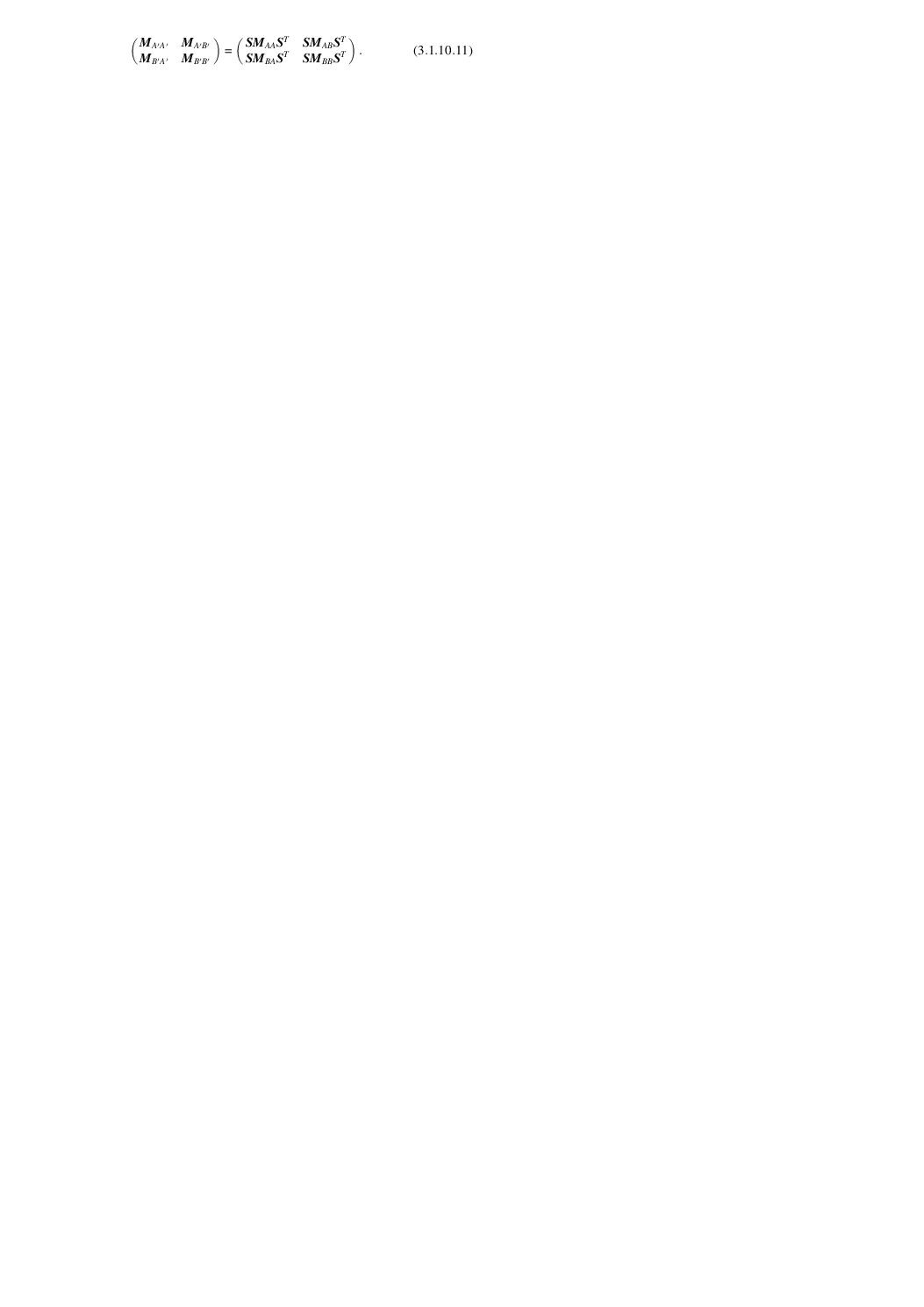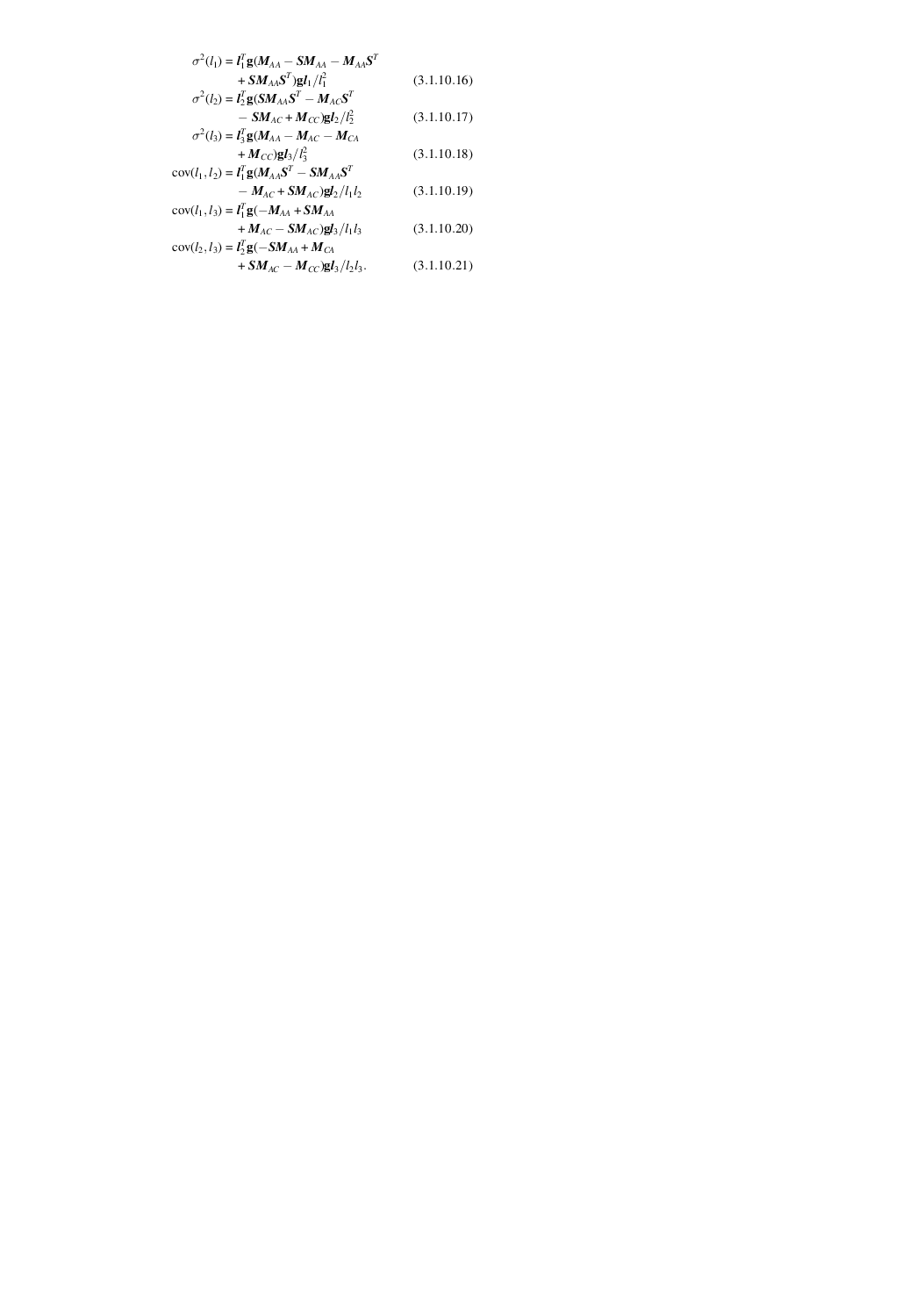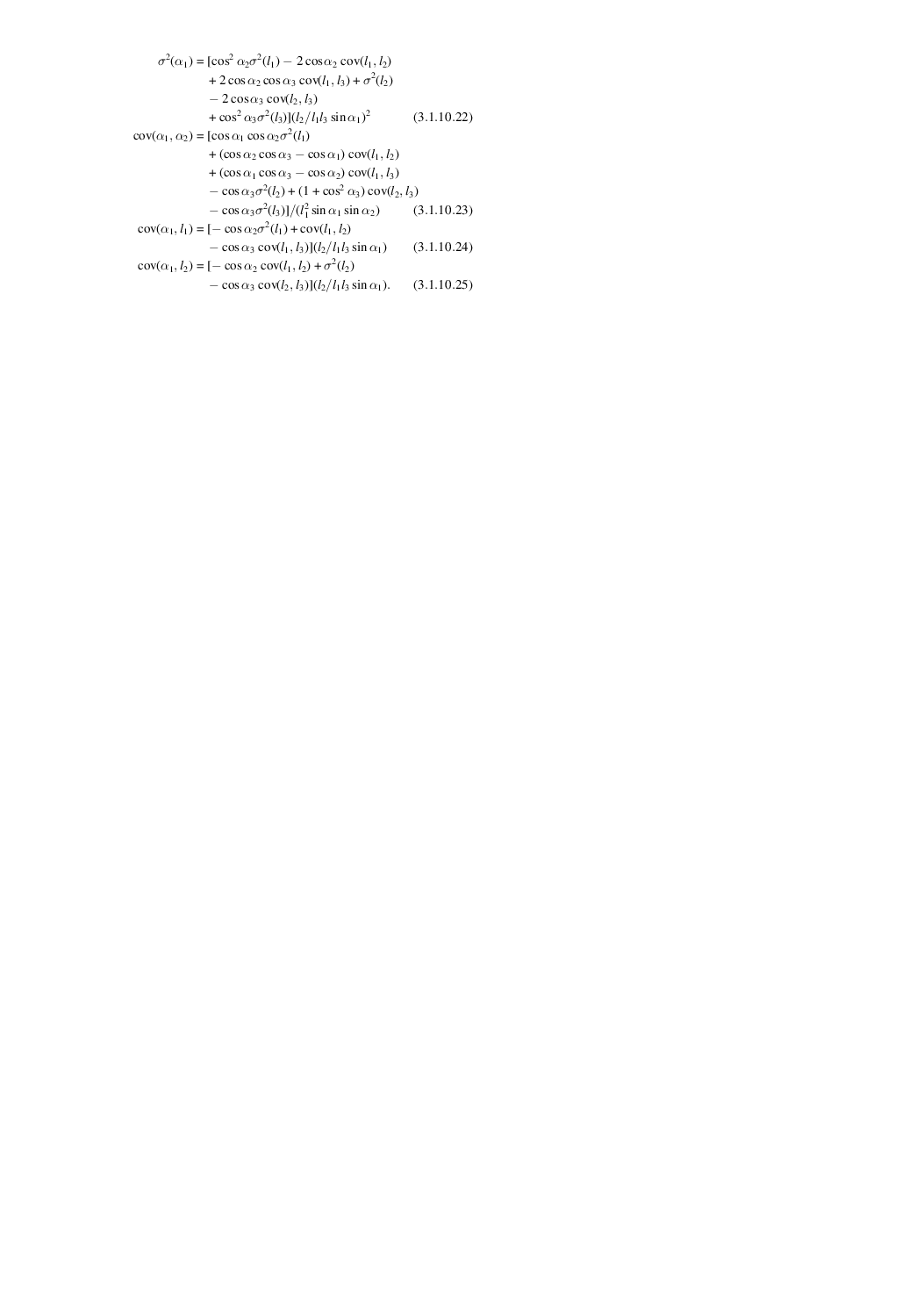International
Tables for
Crystallography
Volume B
Reciprocal space
Edited by U. Shumeli

International Tables for Crystallography (2006). Vol. B, ch. 3.1, pp. 350-351   | 1 | 2 |

## Section 3.1.10. Variance–covariance matrices

D. E. Sandsa*

aDepartment of Chemistry, University of Kentucky, Chemistry–Physics Building, Lexington, Kentucky 40506-0055, USA
Correspondence e-mail: sands@pop.uky.edu

### 3.1.10. Variance–covariance matrices

| top | pdf |

Refinement of a crystal structure yields both the parameters that describe the structure and estimates of the uncertainties of those parameters. Refinement by the method of least squares minimizes a weighted sum of squares of residuals. In the matrix notation of Hamilton's classic book (Hamilton, 1964), values of the m parameters to be determined are expressed by thecolumn vector X given bywhere F is anmatrix representing the observations (structure factors or squares of structure factors), P is anweight matrix that is proportional to the variance–covariance matrix of the observed F, A is andesign matrix consisting of the derivatives of each element of F with respect to each of the parameters andis the transpose of A. The variance–covariance matrix of the parameters is then given byHere, V is thematrix of residuals, consisting of the differences between the observed and calculated values of the elements of F. Sinceis just a single number, M is proportional to the inverse least-squares matrix.

Once the variance–covariance matrix of the parameters is known, the variances and covariances of any quantities derived from these parameters can be computed. The variance of a single function f is given bywhere, as usual, we are using the summation convention and summing over all parameters included in f. A generalization of (3.1.10.3)for two functions is[The covariance of two quantities is, of course, just the variance if the two quantities are the same. For an elementary discussion of statistical covariance and correlation, see Sands (1977).] Equation (3.1.10.4)may now be extended to any number of functions (Sands, 1966); thevariance–covariance matrix C of k functions of m parameters is given in terms of thevariance–covariance matrix of the parameters byin which the ijth element of thematrix D is the derivative of functionwith respect to parameter j. Element(no summation implied over I) is the variance of function, andis the covariance of functionsand.

The calculation of C must, of course, include the contributions of all sources of error, so M in (3.1.10.5)should include the variances and covariances of the unit-cell dimensions and of any other relevant parameters with non-negligible uncertainties.

It may be easier, in some cases, to carry out calculations of variances and covariances in steps. For example, the variance–covariance matrix of a set of distances may be computed and then other quantities may be determined as functions of the distances. It is imperative that all non-vanishing covariances be included in every stage of the calculation; only in special cases are the covariances negligible, and often they are large enough to affect the results seriously (Sands, 1977).

These principles may be used to explore the effects of symmetry or of transformations on the variance–covariance matrices of atomic parameters and derived quantities. Using the notation of Sands (1966), withandthe positional parameters i of atoms A and B, respectively, we defineandasmatrices with ijth elements,,and, respectively. If atomis generated from atom B by symmetry operator S, such thatit is shown in Sands (1966) that the variance–covariance matrices involving atomareIf symmetry operator S is applied to both atoms A and B to generate atomsand, the corresponding matrices may be expressed by the matrix equationIf G is a matrix that transforms to a new set of axes,the transformed variance–covariance matrix of the atomic parameters isTo apply these formulae to calculations of the errors and covariances of interatomic distances and angles, consider the triangle of atoms A, B, C with edges,,, and angles,,at A, B, C, respectively. If the atoms are not related by symmetry,If atom B is generated from atom A by symmetry matrix S, the results, as derived in Sands (1966), areIn equations (3.1.10.14)–(3.1.10.21),is a column vector with components the differences of the coordinates of the atoms connected by the vector. Representative formulae involving the angles,,areIf any of the angles approachor, the denominators in (3.1.10.22)–(3.1.10.25)will become very small, necessitating high-precision arithmetic. Indeterminacies resulting from special relationships between atomic positions may require rederivation of the equations for variances and covariances, to take the relationships into account explicitly and avoid the indeterminacies. A true symmetry condition requiring, for example, a linear bond should cause little problem, and the corresponding variance will be zero. It is the indeterminacies not originating from crystal symmetry that demand caution, in recognizing them and in coping with them correctly.

A general expression for the variance of a dihedral angle, in terms of the variances and covariances of the coordinates of the four atoms, is (Shmueli, 1974)where, in addition to the usual tensor summation over i and j from 1 to 3, summation must be carried out over the four atoms (i.e., k and n vary from 1 to 4). Special cases of (3.1.10.26), corresponding to various levels of approximation of diagonal matrices and isotropic errors, are given in Shmueli (1974). Formulae in dyadic notation are given in Waser (1973)for the variances and covariances of dihedral angles, of best planes, of torsion angles, and of other molecular parameters.

### References

Hamilton, W. C. (1964). Statistics in physical science. New York: Ronald Press.
Sands, D. E. (1966). Transformations of variance–covariance tensors. Acta Cryst. 21, 868–872.
Sands, D. E. (1977). Correlation and covariance. J. Chem. Educ. 54, 90–94.
Shmueli, U. (1974). On the standard deviation of a dihedral angle. Acta Cryst. A30, 848–849.
Waser, J. (1973). Dyadics and the variances and covariances of molecular parameters, including those of best planes. Acta Cryst. A29, 621–631.